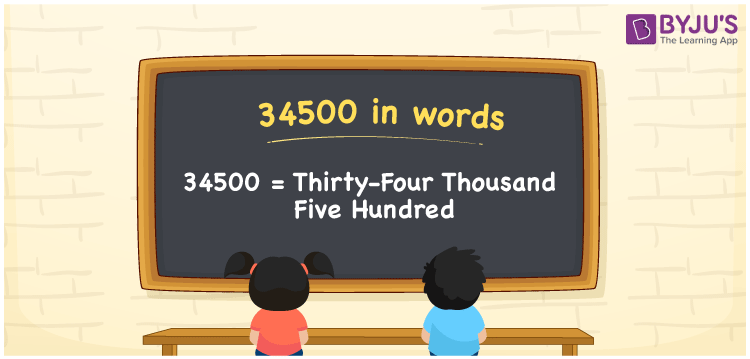# 34500 in Words

34500 in words is written as Thirty-four thousand five hundred. In both the International System of Numerals and the Indian System of Numerals, 34500 in words is written as Thirty-four thousand five hundred. The number 34500 is a Cardinal Number as it represents some quantity. For example, “he has 34500 rupees in his bank account”.

 34500 in words Thirty-four thousand five hundred Thirty-four thousand five hundred in number 34500

## 34500 in English Words

34500 in English words is read as “Thirty-four thousand five hundred.”## How to Write 34500 in Words?

To write 34500 in words, we shall use the place value chart. In the place value chart, put 3 in the ten thousands, 4 in the thousands, 5 in the hundreds, and 0 in the tens and the ones, respectively. Let us make a place value chart to write the number 34500 in words.

 Ten Thousands Thousands Hundreds Tens Ones 3 4 5 0 0

Thus, we can write the expanded form as

3 × Ten Thousand + 4 × Thousand + 5 × Hundred + 0 × Ten + 0 × One

= 3 × 10000 + 4 × 1000 + 5 × 100 + 0 × 10 + 0 × 1

= 30000 + 4000 + 500 + 0 + 0

= 34500

= Thirty-four thousand five hundred.

34500 is a natural number that lies between 34499 and 34501.

34500 in words – Thirty-four thousand five hundred

• Is 34500 an odd number? – No
• Is 34500 an even number? – Yes
• Is 34500 a perfect square number? – No
• Is 34500 a perfect cube number? – No
• Is 34500 a prime number? – No
• Is 34500 a composite number? – Yes

## Frequently Asked Questions on 34500 in Words

Q1

### How to write 34500 in words?

34500 in words is written as Thirty-four thousand five hundred.
Q2

### How to write 34500 in the International and Indian System of Numerals?

In both, the system of numerals, 34500 in words, is written as Thirty-four thousand five hundred.
Q3

### How to write 34500 in a place value chart?

In the place value chart, write 3 in the ten thousands, 4 in the thousands, 5 in the hundreds, and 0 in the tens and the ones, respectively.